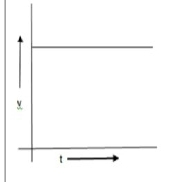"
">

# From the given $v-t$ graph, it can be inferred that the object is(a) At rest(b) In uniform motion(c) Moving with uniform acceleration(d) In non-uniform motion"

In the given $v-t$ graph, it is a straight line parallel to $t-axis$ which indicates that the velocity is increasing with the equal time interval. So the object is moving with uniform acceleration.

So, the option $(c)$ is correct.

Updated on: 10-Oct-2022

35 Views